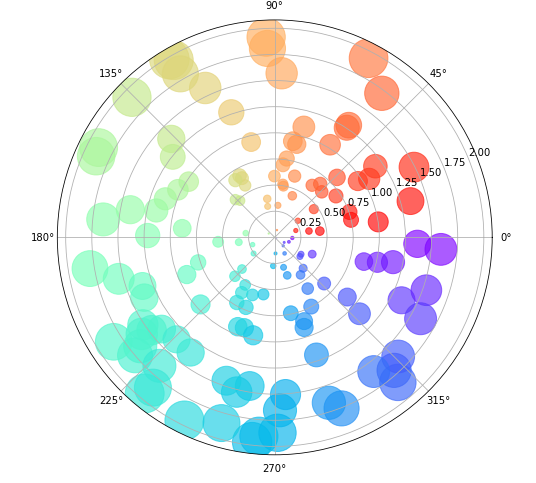# Matplotlib Scatter Plot – Complete Tutorial for Beginners

## Introduction

A lot of times we are required to present results in the form of visualizations that are easy to build and conveys a good amount of information. In this article, we will go through Matplotlib scatter plot tutorial, with practical hands-on of creating different types of scatter plots with several features. We will cover those examples of scattere plot in matplotlib that you may not have usually seen.

### Importing Matplotlib Library

Before starting to plot different values, we’ll need the Matplotlib Library. So let’s import Matplotlib

In :
import numpy as np
import matplotlib.pyplot as plt


## Matplotlib Scatter Plot

A Scatter Plot is used for plotting two different sets of values, helping in finding out correlation amongst the values.

The following section tells about the syntax of the scatter plot function.

### Syntax

matplotlib.pyplot.scatter(x, y, s=None, c=None, marker=None, cmap=None, norm=None, vmin=None, vmax=None, alpha=None, linewidths=None edgecolors=None, *, plotnonfinite=False, data=None, **kwargs)

• x,y : Float or array-like, shape(n,) – These are the two sets of values provided to the scatter function for plotting.
• s : Float or array-like, shape(n,) – This parameter specifies the size of the marker
• c : Array-like or List of Color or Color – This specifies the color of the marker
• marker : MarkerStyle – For setting the marker style, this parameter comes handy
• cmap : str or Colormap, default: ‘viridis’ – Used when we provide c an array of floats
• norm : Normalize, default: None – It helps in normalization of color data for the c.
• vmin, vmax : Float, default: None – When norm is given these parameters aren’t used, but otherwise they help in mapping of color array c to colormap cmap.
• alpha : Float, default: None – It’s a blending value where the range is between 0(transparent) and 1(opaque).
• lindwidths : Float or array-like, default:1.5 – The linewidth of marker is set using this parameter.
• edgecolors : {‘face’,’none’, None} or Color or Color Sequence – The edge color of the marker is set with this parameter. With ‘face’, the edge color will always be same as face color. With ‘none’, No patch boundary will be drawn.

The function returns a plot with desired axes and other parameters.

### Example 1: Simple Scatter Plot

This first example is a simple scatter plot that uses randomly generated data.

The data is generated using numpy’s random seed function which helps in generating pseudo numbers.

The color and size of the markers(dots) is decided by the random number they represent.

The scatter function is provided with the data points through ‘x’ and ‘y’ parameter. The ‘s’ and ‘c’ parameters specify the size and color of the markers. Lastly, the ‘alpha’ parameter is used for increasing the transparency of the markers. The range of alpha parameter ranges from 0 to 1.

The cmap or colormap parameter is used for giving different colors to the markers.

In :
np.random.seed(11808096)

N = 75
x = np.random.rand(N)
y = np.random.rand(N)
colors = np.random.rand(N)
area = (25 * np.random.rand(N))**2

plt.scatter(x, y, s=area, c=colors, cmap = 'Spectral_r', alpha=0.9)
plt.show()

Output: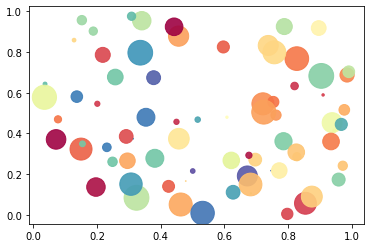### Example 2: Scatter Plot Masked

In this sort of example, some of the data points are masked(hidden) and there is a line been made for demarking the masked regions.

In masked scatter plot, we create a boundary in which the data points are represented differently (masked) and other data points are represented differently.

The scatter function is called for both the areas. To make the two regions standout, there is a boundary created with the help of plt.plot function.

To determine the angle of the boundary, numpy’s arange function is used.

In :
np.random.seed(11808096)

N = 75
r0 = 0.5
x = 0.9 * np.random.rand(N)
y = 0.9 * np.random.rand(N)
area = (30 * np.random.rand(N))**2
c = np.sqrt(area)
r = np.sqrt(x ** 2 + y ** 2)

# Defining the two different areas
area1 = np.ma.masked_where(r < r0, area)
area2 = np.ma.masked_where(r >= r0, area)
plt.scatter(x, y, s=area1, marker='o', c=c, cmap= 'gist_rainbow_r')
plt.scatter(x, y, s=area2, marker='*', c=c, cmap= 'tab20_r')

# Code for building a boundary between the regions
theta = np.arange(0, np.pi / 2, 0.01)
plt.plot(r0 * np.cos(theta), r0 * np.sin(theta))

plt.show()

Output: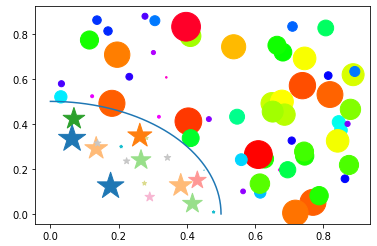### Example 3: Scatter Plot with Pie Chart Markers

In this type of scatter plot, the markers are made in the form of pie chart that depict an extra information about the data.

For the 3rd example, we’ll be combining two different plots into a single plot for conveying more information. In the previous example, the markers made for scatter plots were simple dots that presented a value, but here the markers is represented with the help of pie charts.

Initially, we define the ratios for the radii of the pie chart markers. After this, we randomly provide the relative sizes for the pie charts in the form of an array. After this, the pie chart sections are created with the help of numpy’s sin and cos functions.

The penultimate step is to call the subplots function for plotting the pie chart along with the scatter plot. At last, the scatter function is called for building the scatter plot with pie charts as markers.

In :
# Defining the ratios for radius of pie chart markers
r1 = 0.2       # 20%
r2 = r1 + 0.2  # 40%
r3 = r2 + 0.4  # 80%

# define some sizes of the scatter marker
sizes = np.array([45, 90, 135, 180, 225, 270, 315, 360])

# calculate the points of the first pie marker
# these are just the origin (0, 0) + some (cos, sin) points on a circle
x1 = np.cos(2 * np.pi * np.linspace(0, r1))
y1 = np.sin(2 * np.pi * np.linspace(0, r1))
xy1 = np.row_stack([[0, 0], np.column_stack([x1, y1])])
s1 = np.abs(xy1).max()

x2 = np.cos(2 * np.pi * np.linspace(r1, r2))
y2 = np.sin(2 * np.pi * np.linspace(r1, r2))
xy2 = np.row_stack([[0, 0], np.column_stack([x2, y2])])
s2 = np.abs(xy2).max()

x3 = np.cos(2 * np.pi * np.linspace(r2, r3))
y3 = np.sin(2 * np.pi * np.linspace(r2, r3))
xy3 = np.row_stack([[0, 0], np.column_stack([x3, y3])])
s3 = np.abs(xy3).max()

x4 = np.cos(2 * np.pi * np.linspace(r3, 1))
y4 = np.sin(2 * np.pi * np.linspace(r3, 1))
xy4 = np.row_stack([[0, 0], np.column_stack([x4, y4])])
s4 = np.abs(xy4).max()

fig, ax = plt.subplots()
ax.scatter(range(8), range(8), marker=xy1, s=s1**2 * sizes, facecolor='blue')
ax.scatter(range(8), range(8), marker=xy2, s=s2**2 * sizes, facecolor='green')
ax.scatter(range(8), range(8), marker=xy3, s=s3**2 * sizes, facecolor='red')
ax.scatter(range(8), range(8), marker=xy4, s=s3**2 * sizes, facecolor='orange')

plt.show()

Output: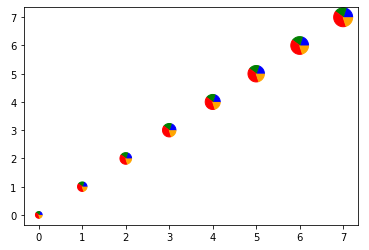### Example 4: Scatter Plot with different marker style

Here in this example, a different type of marker will be used in the plot.

The fourth example of this matplotlib tutorial on scatter plot will tell us how we can play around with different marker styles.

Here in this example, we have used two different marker styles. You can explore various types of markers from here.

We have to use the $symbol to specify the type of marker we desire in our scatter plot. Apart from this, we are using numpy’s arange and random function for generating pseudo numbers for plotting scatter plot. The color of the two markers are passed in the c parameter and alpha parameter ensures that the transparency level is correct. In : np.random.seed(11808096) x1 = np.arange(0.0, 50.0, 2.0) y1 = x1 ** 1.3 + np.random.rand(*x1.shape) * 30.0 s1 = np.random.rand(*x1.shape) * 800 + 500 x2 = np.arange(0.0, 50.0, 2.0) y2 = x2 ** 0.3 + np.random.rand(*x2.shape) * 25.0 s2 = np.random.rand(*x2.shape) * 800 + 500 plt.scatter(x1, y1, s1, c="g", alpha=0.7, marker=r'$\star$',label="Fortune") plt.scatter(x2, y2, s2, c="r", alpha=0.8, marker=r'$\diamondsuit\$',label="Fortune")

plt.xlabel("Area Covered")
plt.ylabel("Diamnonds and Stars Found")
plt.legend(loc='upper left')
plt.show()

Output: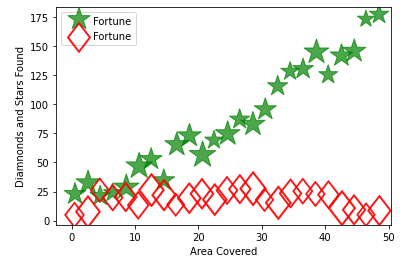### Example 5: Scatter Plots on a Polar Axis

In the case of polar axis, the size of the marker increases radially, and also the color increases with an increase in angle.

The last example of this matplotlib scatter plot tutorial is a scatter plot built on the polar axis. Polar axes are generally different from normal axes, here in this case we have the liberty to place the values across 360 degrees.

After specifying the count of markers with the parameter “N”, we will be assigning radius, angle, area, and colors with the help of the random function of numpy.

Through figure function, we are able to print the plot, and figsize help in increasing or decreasing the size of plot. Subplot function helps in plotting the scatter plot over polar axis.

Lastly, scatter function is called where we also specify the cmap or color map parameter for assigning different colors to the markers.

In :
np.random.seed(11808096)

# Compute areas and colors
N = 150
r = 2 * np.random.rand(N)
theta = 2 * np.pi * np.random.rand(N)
area = 400 * r**2
colors = theta

fig = plt.figure(figsize=(10,8))